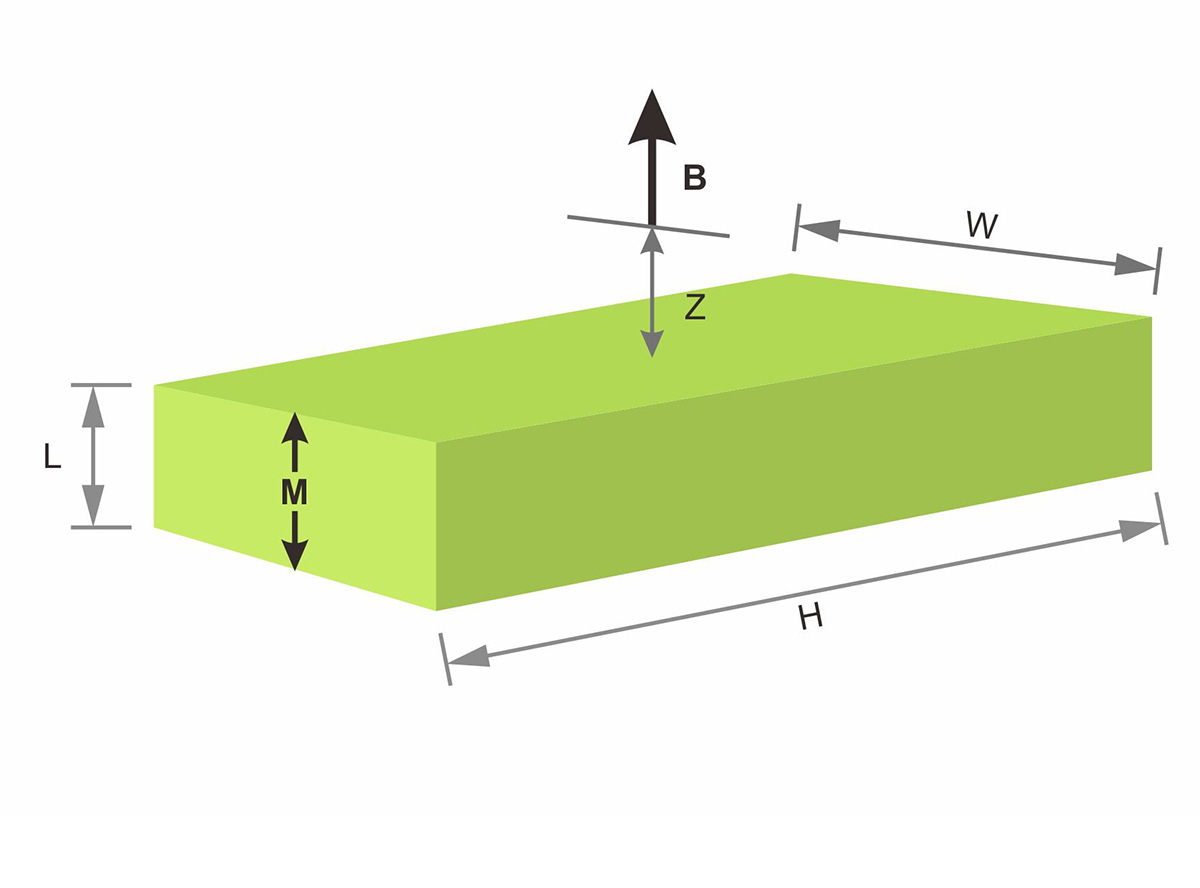# Calculator for Flux Density

Magnetic flux density or magnetic field strength for one magnet is easy for magnet users to get a general idea of the magnet strength. In many cases they expect to get the magnet strength data before measuring the actual magnet sample via the instrument, like Tesla Meter, Gauss Meter, etc. Horizon Magnetics hereby prepare a simple calculator for you to calculate flux density conveniently. Flux density, in gauss, can be calculated at any distance from the end of a magnet. Results are for field strength on-axis, at a distance "Z" from a pole of the magnet. These calculations only work with "square loop" or "straight line" magnetic materials such as Neodymium, Samarium Cobalt and Ferrite magnets. They should not be used for Alnico magnet.
Flux Density of a Cylindrical MagnetTotal Air Gap > 0
Z =mm
Magnet Length
L =mm
Diameter
D =mm
Residual Induction
Br =Gauss
Result
Flux Density
B =Gauss
Flux Density of a Rectangular MagnetTotal Air Gap > 0
Z =mm
Magnet Length
L =mm
Width
W =mm
Height
H =mm
Residual Induction
Br =Gauss
Result
Flux Density
B =Gauss
Accuracy Statement

The result of flux density is calculated in theory and it might have some percentages of deviation from the actual measuring data. Although we make every effort to ensure that the calculations above are complete and accurate, we make no warranty regarding them. We would appreciate your input, so contact us regarding corrections, additions and suggestions for improvement.